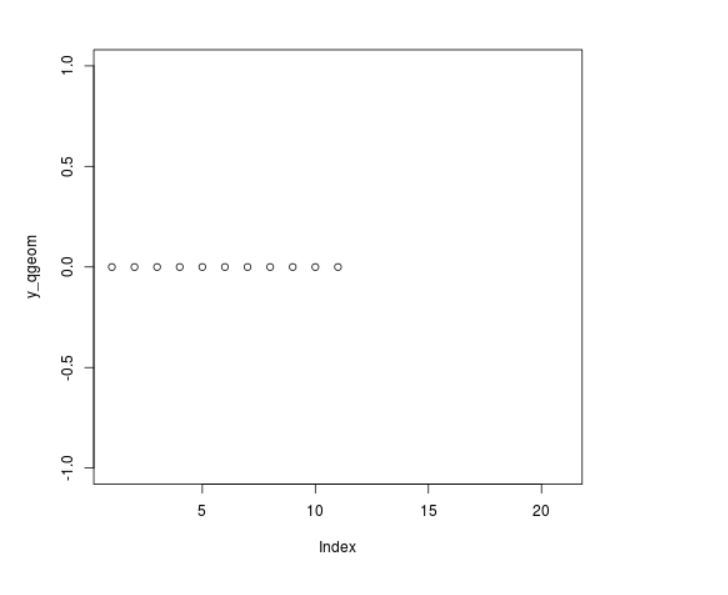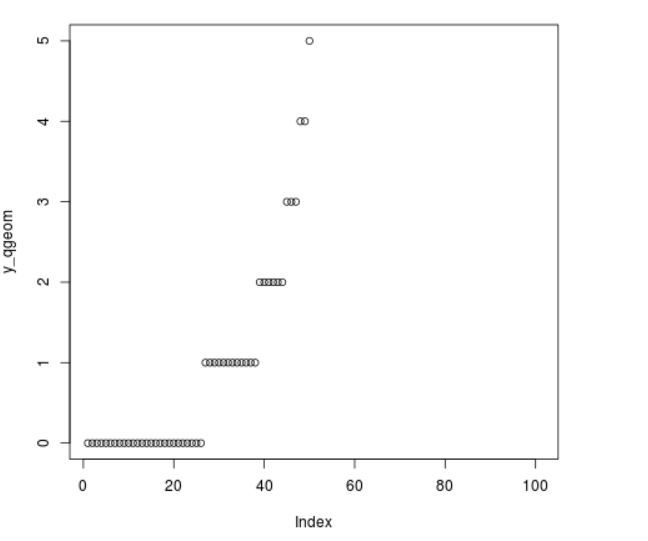# Compute the Value of Geometric Quantile Function in R Programming – qgeom() Function

`qgeom()` Function in R Language is used to plot a graph for quantile function of Geometric Distribution.

Syntax: qgeom(x, prob)

Parameters:
x: x-values of qgeom() function
prob: prob of plot

Example 1:

 `# R program to illustrate ` `# qgeom() function in r ` ` `  `# Specify x-values for qgeom function ` `x_qgeom <``-` `seq(``0``, ``2``, by ``=` `0.1``)    ` ` `  `# Apply qgeom function            ` `y_qgeom <``-` `qgeom(x_qgeom, prob ``=` `1``)  ` `# Plot qgeom values     ` `plot(y_qgeom)                                                   `

Output:Example 2:

 `# R program to illustrate ` `# qgeom() function in r ` ` `  `# Specify x-values for qgeom function ` `x_qgeom <``-` `seq(``0``, ``2``, by ``=` `0.02``)    ` ` `  `# Apply qgeom function            ` `y_qgeom <``-` `qgeom(x_qgeom, prob ``=` `0.5``)  ` `# Plot qgeom values     ` `plot(y_qgeom)  `

Output:My Personal Notes arrow_drop_upCheck out this Author's contributed articles.

If you like GeeksforGeeks and would like to contribute, you can also write an article using contribute.geeksforgeeks.org or mail your article to contribute@geeksforgeeks.org. See your article appearing on the GeeksforGeeks main page and help other Geeks.

Please Improve this article if you find anything incorrect by clicking on the "Improve Article" button below.

Article Tags :

Be the First to upvote.

Please write to us at contribute@geeksforgeeks.org to report any issue with the above content.